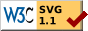# Learnt Laurent Series

Yesterday, I read the proof of Laurent’s Theorem in my complex variables book, and I was stuck at the following equation.1

Suppose that $f$ is analytic on the annular region $R_1 < \abs{z - z_0} < R_2$. Let $C$ be any postively-oriented closed curve surrounding point $z_0$. By Cauchy–Goursat Theorem, we have

$f(z) = \sum_{n = -\infty}^{\infty} c_n (z - z_0)^n \quad (R_1 < \abs{z - z_0} < R_2).$

Image size: 300Source code: $\rm \LaTeX$, SVG

I was stuck at this point.

\begin{equation} \int_{C_2}^{} \frac{f(s) \ud s}{s - z} - \int_{C_1}^{} \frac{f(s) \ud s}{s - z} - \int_{\gamma}^{} \frac{f(s) \ud s}{s - z} = 0 \label{int} \end{equation}

The function $f$ is analytic on the entire circle $\gamma$, including the circumference of $\gamma$. Then the boundary of the region where $f$ is analytic is the circumferences of $C_1$ and $C_2$. I wondered why the third term in $\eqref{int}$ was needed. I took me half an hour to figure out that the integrand is not simply $f(s)$, but with a denominator $s - z$. Since $z$ is the centre of the circle $\gamma$, I need to also include it as the boundary of the region of analyticity of the integrand.

1. Brown, J. W., Churchill, R. V., & Lapidus, M. (1996). Complex variables and applications (Vol. 7). (pp. 17). New York: McGraw-Hill.